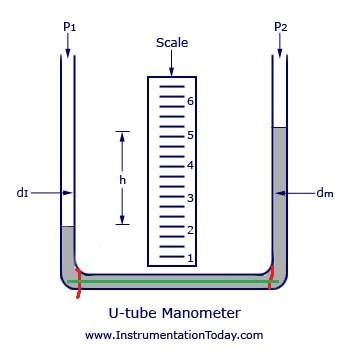# Is the pressure equal everywhere here?

anonymous99
Homework Statement:
Consider the picture below. I know the pressure is constant across the green line because pressure is fixed at a given depth. However, assume the red lines are physical boundaries. Would the pressure still be constant across the green line? My reasoning is no because inside the two red line boundaries, there is a smaller layer of water above so less pressure from the weight of thee water above whereas at the left/right sides there is a larger layer so more pressure. But if the pressure is unequal at either side of the red line, then the boundary should move until pressure equalizes so is my reasoning incorrect?
Relevant Equations:
sGold Member
2022 Award
what kind of physical boundaries? You imply they can move but that's not much of a description.

anonymous99
I was thinking like a piston in a cylinder.

Gold Member
2022 Award
I was thinking like a piston in a cylinder.
Well, if it's completely moveable (no friction) then it is irrelevant to the description of pressure and can be left out of the discussion.

•Chestermiller
anonymous99
Well, if it's completely moveable (no friction) then it is irrelevant to the description of pressure and can be left out of the discussion.
I see. Is that because it would just end up moving until pressure is equalized? What if it was a fixed boundary. Then pressure must be unequal on both sidies right?

Mentor
If the system is in static equilibrium, the pressure does not vary horizontally, irrespective of any boundaries.

anonymous99
If the system is in static equilibrium, the pressure does not vary horizontally, irrespective of any boundaries.
Hmm. But how would the pressure be equal if the weight of water above is different inside and outside the boundary? Can they not be considered as two separate systems now.

Gold Member
2022 Award
@Anonymous 69 it may just be me but I find your question(s) ill formed. You seem to keep jumping from on scenario to another but considering them the same. Try to describe ONE scenario, and be VERY precise about the conditions and exactly what it is you are confused about.

•PhDeezNutz and etotheipi
Mentor
Hmm. But how would the pressure be equal if the weight of water above is different inside and outside the boundary? Can they not be considered as two separate systems now.
Have you drawn a free body diagram of the water in the horizontal section, or do you feel that you’ve advanced beyond the need to use free body diagrams?

anonymous99
Have you drawn a free body diagram of the water in the horizontal section, or do you feel that you’ve advanced beyond the need to use free body diagrams?
Yes and from my FBD it seems the pressure is different but I think I might be wrong. At a certain point on the green line inside the boundaries, the fluid particle experiences a force equal to the weight of the water above and this is balanced by an equal force from below. Since pressure is isotropic, forces in the x-direction on the particle are equal to this force too. Now, for a point on the same line at the left/right sides of the manometer, there is a larger weight of water above and thus more force = more presssure.

Mentor
What does your horizontal force balance tell you?

•